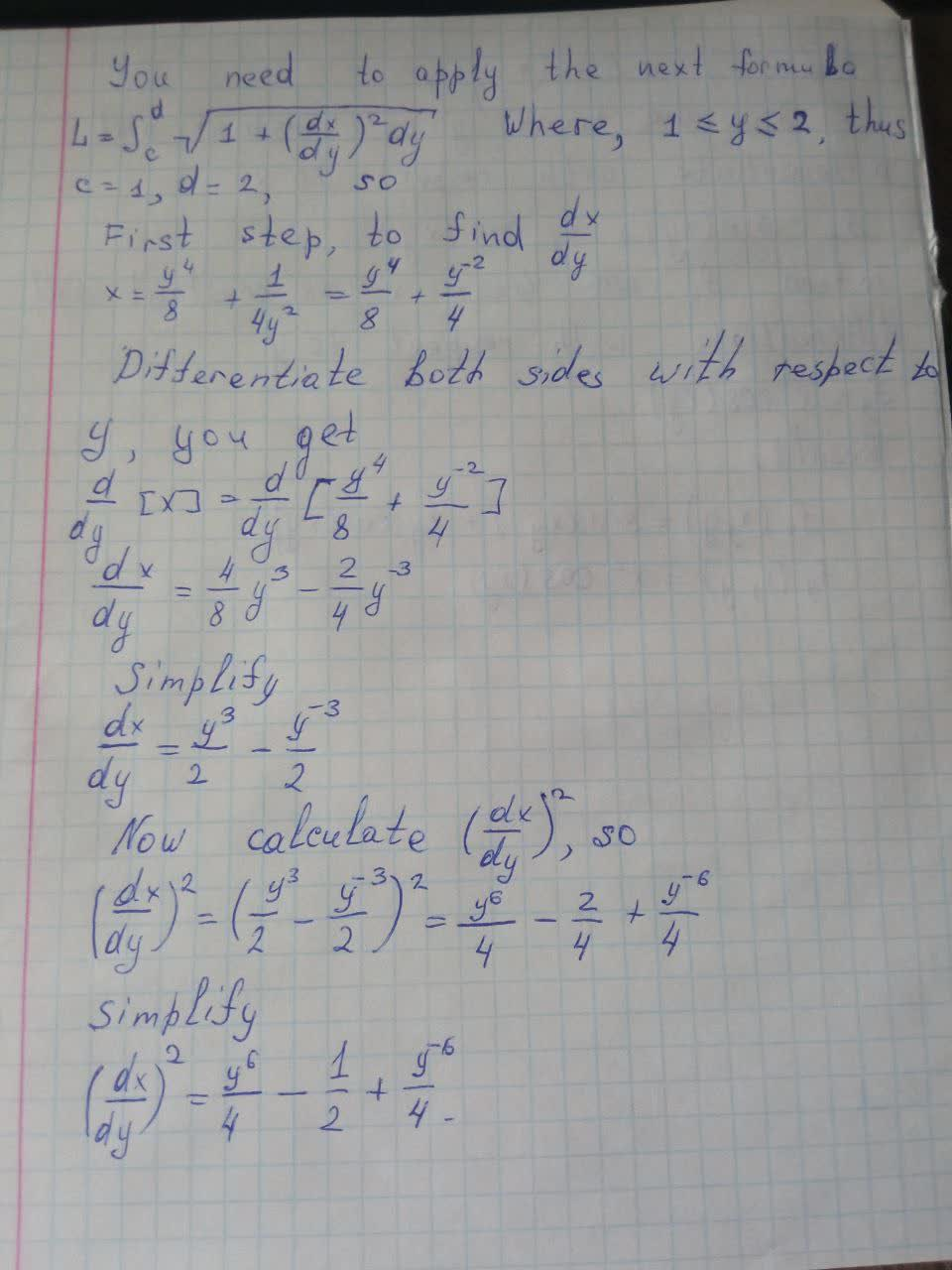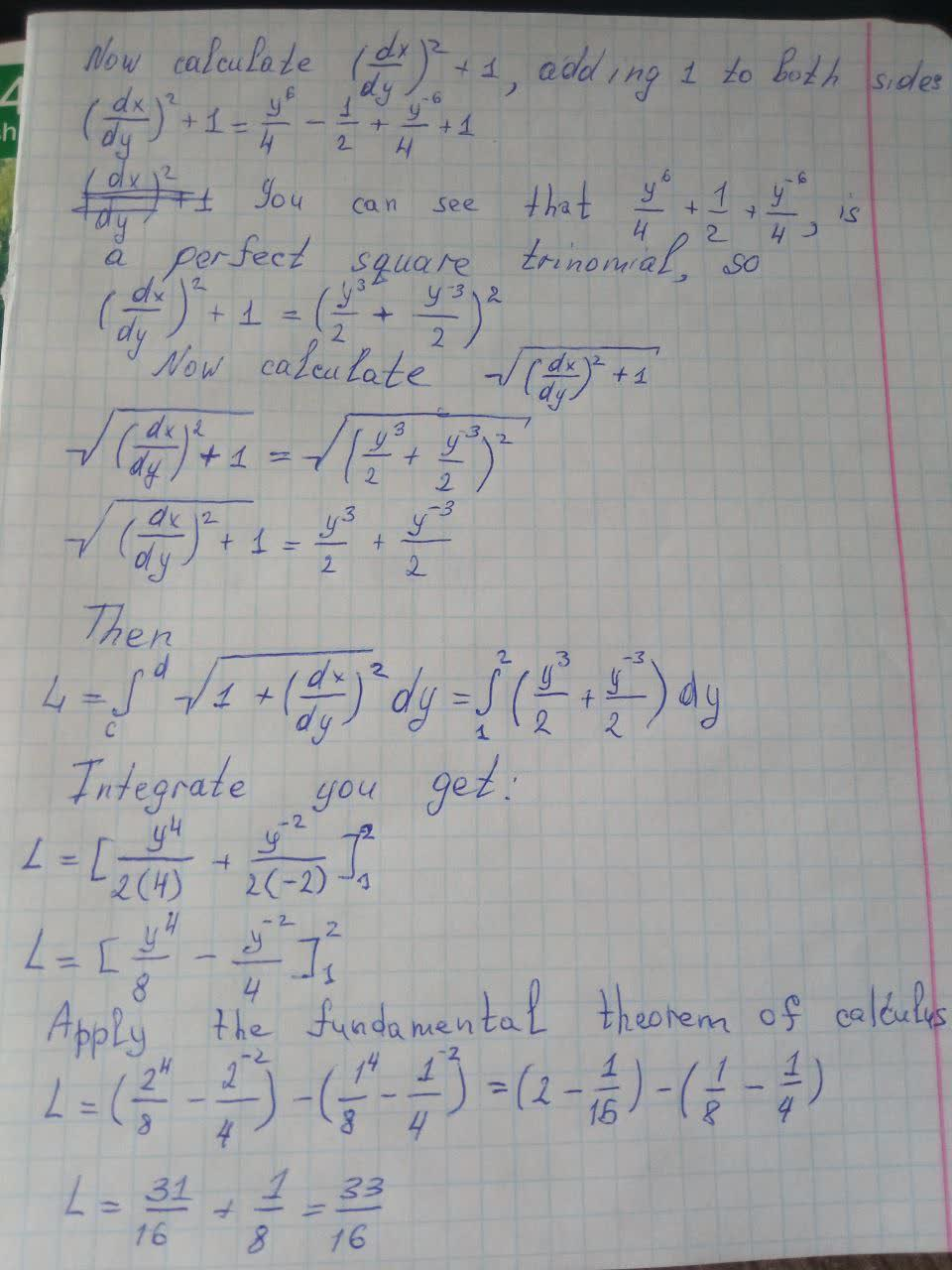# Find the exact length of the curve x=\frac{y^4}{8}+\frac{1}{4y^2}\quad1\leq y\leq2Dottie Parra 2021-05-18 Answered
Find the exact length of the curve
$x=\frac{{y}^{4}}{8}+\frac{1}{4{y}^{2}}\phantom{\rule{1em}{0ex}}1\le y\le 2$
You can still ask an expert for help

• Questions are typically answered in as fast as 30 minutes

Solve your problem for the price of one coffee

• Math expert for every subject
• Pay only if we can solve itYusuf Keller